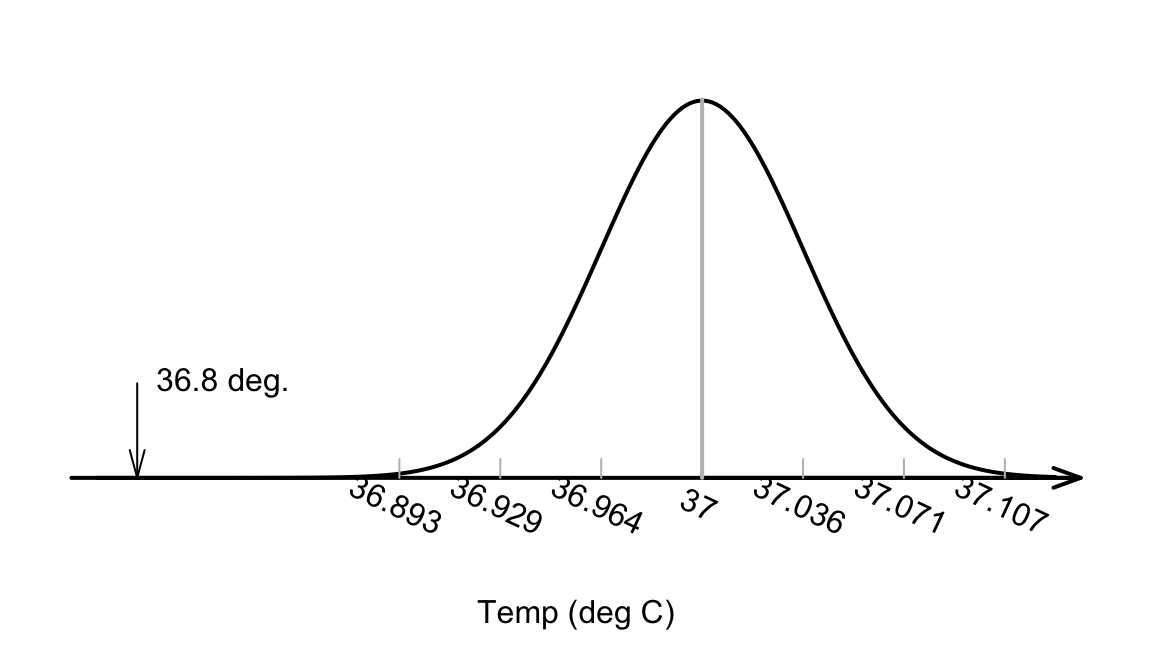## 27.4 The test statistic and $$t$$-scores: One mean

The sampling distributions describes what to expect from the sample mean, assuming $$\mu = 37.0^\circ\text{C}$$. The value of $$\bar{x}$$ that is observed, however, is $$\bar{x}=36.8051^\circ$$ How likely is it that such a value could occur by chance?

The value of the observed sample mean can be located the picture of the sampling distribution (Fig. 27.3). The value $$\bar{x}=36.8051^\circ\text{C}$$ is unusually small. About how many standard deviations is $$\bar{x}$$ away from $$\mu=37$$? A lot…FIGURE 27.3: The sample mean of $$\bar{x}=36.8041^\circ$$C is very unlikely to have been observed if the poulation mean really was $$37^\circ$$C, and $$n=130$$

Relatively speaking, the distance that the observed sample mean (of $$\bar{x}=36.8051$$) is from the mean of the sampling distribution (Fig. 27.3). is found by computing how many standard deviations the value of $$\bar{x}$$ is from the mean of the distribution; that is, computing something like a $$z$$-score. (Remember that the standard deviation in Fig. 27.3 is the the standard error: the amount of variation in the sample means.)

Since the mean and standard deviation (i.e., the standard error) of this normal distribution are known, the number of standard deviations that $$\bar{x} = 36.8051$$ is from the mean is

$\frac{36.8051 - 37.0}{0.035724} = -5.453.$ This value is like a $$z$$-score. However, this is actually called a $$t$$-score because it has been computed when the population standard deviation is unknown, and the best estimate (the sample standard deviation) is used when $$\text{s.e.}(\bar{x})$$ was computed.

Both $$t$$ and $$z$$ scores measure the number of standard deviations that an observation is from the mean: $$z$$-scores use $$\sigma$$ and $$t$$-scores use $$s$$. Here, the distribution of the sample statistic is relevant, so the appropriate standard deviation is the standard deviation of the sampling distribution: the standard error.

Like $$z$$-scores, $$t$$-scores measure the number of standard deviations that an observation is from the mean. $$z$$-scores are calculated using the population standard deviation, and $$t$$-scores are calculated using the sample standard deviation.

In hypothesis testing, $$t$$-scores are more commonly used than $$z$$-scores, because almost always the population standard deviation is unknown, and the sample standard deviation is used instead.

In this course, it is sufficient to think of $$z$$-scores and $$t$$-scores as approximately the same. Unless sample sizes are small, this is a reasonable approximation.

So the calculation is:

$t = \frac{36.8051 - 37.0}{0.035724} = -5.453;$ the observed sample mean is more than five standard deviation below the population mean. This is highly unusual based on the 68–95–99.7 rule, as seen in Fig. 27.3.

In general, a $$t$$-score in hypothesis testing is

$\begin{equation} t = \frac{\text{sample statistic} - \text{assumed population parameter}} {\text{standard error of the sample statistic}}. \tag{27.1} \end{equation}$